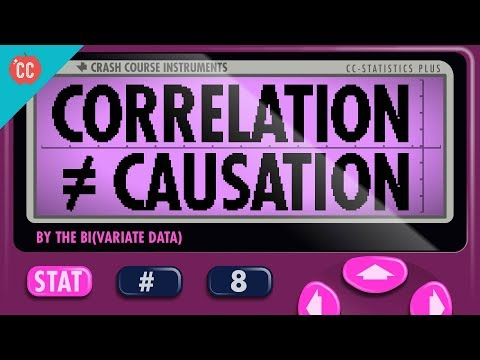### Submit a Resource

Crash Course: Statistics #8 - Correlation Doesn't Equal CausationToday we’re going to talk about data relationships and what we can learn from them.

We’ll focus on correlation, which is a measure of how two variables move together, and we’ll also introduce some useful statistical terms you’ve probably heard of like regression coefficient, correlation coefficient (r), and r^2. But first, we’ll need to introduce a useful way to represent bivariate continuous data – the scatter plot. The scatter plot has been called “the most useful invention in the history of statistical graphics” but that doesn’t necessarily mean it can tell us everything. Just because two data sets move together doesn’t necessarily mean one CAUSES the other. This gives us one of the most important tenets of statistics: correlation does not imply causation.

Data, Graphing & Statistics
High School, College

Crash Course

Video